Next: Wave Pulses Up: Multi-Dimensional Waves Previous: Sound Waves in Fluids

# Exercises

1. Show that the one-dimensional plane wave, (529), is a solution of the one-dimensional wave equation, (536), provided that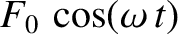Likewise, demonstrate that the three-dimensional plane wave, (533), is a solution of the three-dimensional wave equation, (537), as long as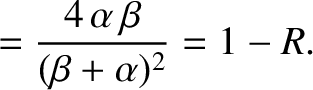2. Demonstrate that for a cylindrically symmetric wavefunction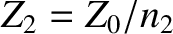, where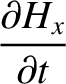, the three-dimensional wave equation (537) can be re-writtenShow that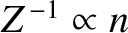is an approximate solution of this equation in the limit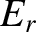, where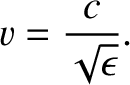.

3. Demonstrate that for a spherically symmetric wavefunction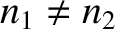, where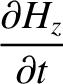, the three-dimensional wave equation (537) can be re-written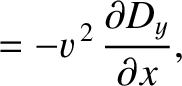Show that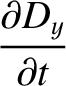is a solution of this equation, where.

4. Consider an elastic sheet stretched over a rectangular frame that extends from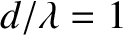to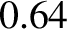, and from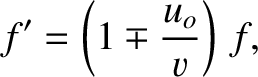to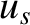. Suppose that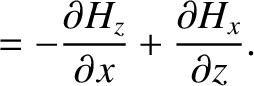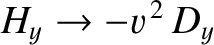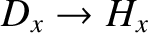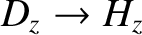Show that the amplitudes and phase angles in the normal mode expansion (556) are given by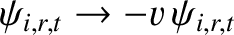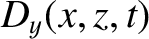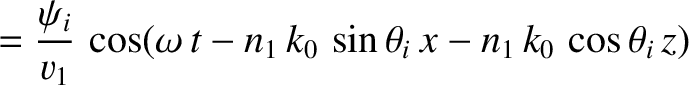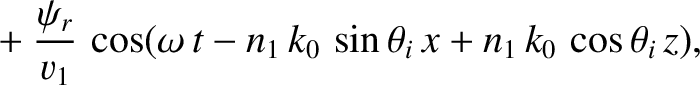where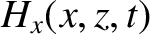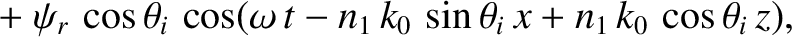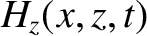5. The radial oscillations of an ideal gas in a spherical cavity of radius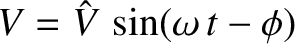are governed by the spherical wave equation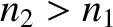subject to the boundary condition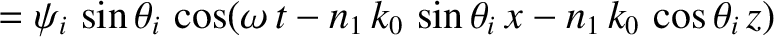. Here,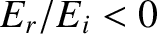is a spherical coordinate,is the radial displacement, andis the speed of sound. Show that the general solution of this equation is written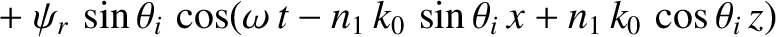where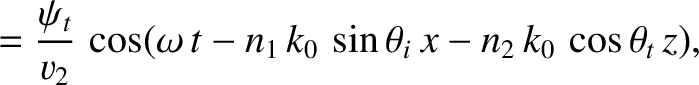,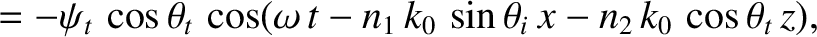and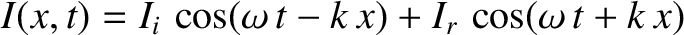,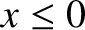are arbitrary constants.

6. Show that a light-ray entering a planar transparent plate of thickness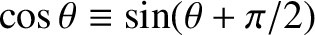and refractive index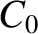emerges parallel to its original direction. Show that the lateral displacement of the ray is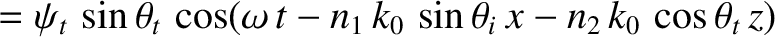where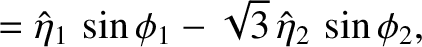and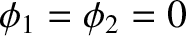are the angles of incidence and refraction, respectively, at the front side of the plate.

7. Suppose that a light-ray is incident on the front (air/glass) interface of a uniform pane of glass of refractive indexat the Brewster angle. Demonstrate that the refracted ray is also incident on the rear (glass/air) interface of the pane at the Brewster angle. [From Fitzpatrick 2008.]

8. Show that the Fresnel relations, (603) and (604), for the polarization in which the magnetic intensities of all three waves are parallel to the interface can be written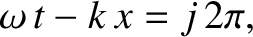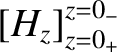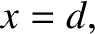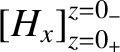where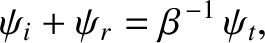represents impedance. (Here,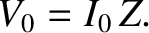is the impedance of free space, andthe refractive index.) Demonstrate that the Fresnel relations, (625) and (626), for the other polarization take the form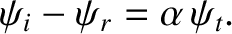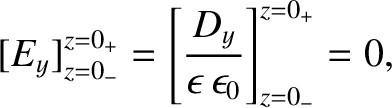9. Show that the expressions, (695) and (696), for the coefficients of reflection and transmission for a sound wave obliquely incident at an interface between two immiscible fluids can be written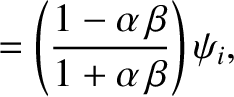where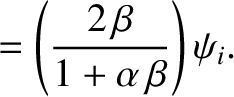and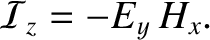are the acoustic impedances of the two fluids. Show that the expression, (697), for the angle of intromission can be written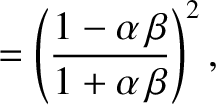Next: Wave Pulses Up: Multi-Dimensional Waves Previous: Sound Waves in Fluids
Richard Fitzpatrick 2013-04-08